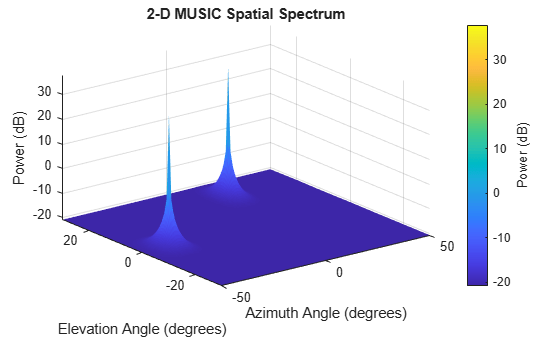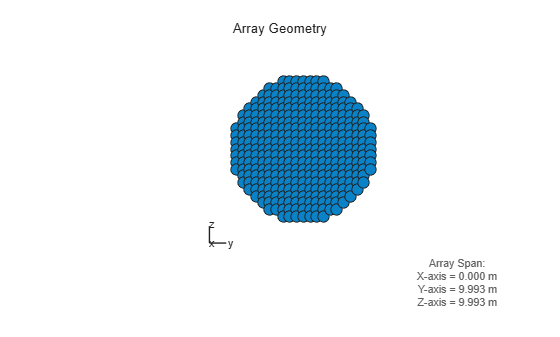phased.MUSICEstimator2D

Estimate 2D direction of arrival using narrowband MUSIC algorithm

Description

The phased.MUSICEstimator2D System object™ implements the narrowband multiple signal classification (MUSIC) algorithm for 2-D planar or 3-D arrays such as a uniform rectangular array (URA). MUSIC is a high-resolution direction-finding algorithm capable of resolving closely-spaced signal sources. The algorithm is based on the eigenspace decomposition of the sensor covariance matrix.

To estimate directions of arrival (DOA):

1. Define and set up a phased.MUSICEstimator2D System object. See Construction.

2. Call the step method to estimate the DOAs according to the properties of phased.MUSICEstimator2D.

Note

Instead of using the step method to perform the operation defined by the System object, you can call the object with arguments, as if it were a function. For example, y = step(obj,x) and y = obj(x) perform equivalent operations.

Construction

estimator = phased.MUSICEstimator2D creates a MUSIC DOA estimator System object, estimator.

estimator = phased.MUSICEstimator2D(Name,Value) creates a System object, estimator, with each specified property Name set to the specified Value. You can specify additional name-value pair arguments in any order as (Name1,Value1,...,NameN,ValueN).

Properties

expand all

Sensor array, specified as a Phased Array System Toolbox array System object.

Example: phased.URA

Signal propagation speed, specified as a real-valued positive scalar. Units are in meters per second. The default propagation speed is the value returned by physconst('LightSpeed').

Example: 3e8

Data Types: single | double

Operating frequency, specified as a positive scalar. Units are in Hz.

Example: 1e9

Data Types: single | double

Enable forward-backward averaging, specified as false or true. Set this property to true to use forward-backward averaging to estimate the covariance matrix for sensor arrays with a conjugate symmetric array manifold.

Data Types: logical

Azimuth scan angles, specified as a or real-valued row vector. Angle units are in degrees. The angle values must lie between –180° and 180°, inclusive, and be in ascending order.

Example: [-30:20]

Data Types: single | double

Elevation scan angles, specified as a real-valued row vector. Angle units are in degrees. The angle values must lie between –90° and 90°, inclusive, and be in ascending order.

Example: [-70:75]

Data Types: single | double

Option to enable directions-of-arrival (DOA) output, specified as false or true. To obtain the DOA of signals, set this property to true. The DOAs are returned in the second output argument when the object is executed.

Data Types: logical

Source of the number of arriving signals, specified as 'Auto' or 'Property'.

• 'Auto' — The System object estimates the number of arriving signals using the method specified in the NumSignalsMethod property.

• 'Property' — Specify the number of arriving signals using the NumSignals property.

Data Types: char

Method used to estimate the number of arriving signals, specified as 'AIC' or 'MDL'.

• 'AIC' — Akaike Information Criterion

• 'MDL' — Minimum Description Length criterion

Dependencies

To enable this property, set NumSignalsSource to 'Auto'.

Data Types: char

Number of arriving signals for DOA estimation, specified as a positive integer.

Example: 3

Dependencies

To enable this property, set NumSignalsSource to 'Property'.

Data Types: single | double

Methods

 plotSpectrum Plot 2-D MUSIC spectrum reset Reset states of System object step Estimate direction of arrival using 2-D MUSIC
Common to All System Objects
release

Allow System object property value changes

Examples

collapse all

Assume that two sinusoidal waves of frequencies 450 Hz and 600 Hz strike a URA from two different directions. Signals arrive from -37° azimuth, 0° elevation and 17° azimuth, 20° elevation. Use 2-D MUSIC to estimate the directions of arrival of the two signals. The array operating frequency is 150 MHz and the signal sampling frequency is 8 kHz.

f1 = 450.0;
f2 = 600.0;
doa1 = [-37;0];
doa2 = [17;20];
fc = 150e6;
c = physconst('LightSpeed');
lam = c/fc;
fs = 8000;

Create the URA with default isotropic elements. Set the frequency response range of the elements.

array = phased.URA('Size',[11 11],'ElementSpacing',[lam/2 lam/2]);
array.Element.FrequencyRange = [50.0e6 500.0e6];

Create the two signals and add random noise.

t = (0:1/fs:1).';
x1 = cos(2*pi*t*f1);
x2 = cos(2*pi*t*f2);
x = collectPlaneWave(array,[x1 x2],[doa1,doa2],fc);
noise = 0.1*(randn(size(x))+1i*randn(size(x)));

Create and execute the 2-D MUSIC estimator to find the directions of arrival.

estimator = phased.MUSICEstimator2D('SensorArray',array,...
'OperatingFrequency',fc,...
'NumSignalsSource','Property',...
'DOAOutputPort',true,'NumSignals',2,...
'AzimuthScanAngles',-50:.5:50,...
'ElevationScanAngles',-30:.5:30);
[~,doas] = estimator(x + noise)
doas = 2×2

-37    17
0    20

The estimated DOAs exactly match the true DOAs.

Plot the 2-D spatial spectrum.

plotSpectrum(estimator);Assume that two sinusoidal waves of frequencies 1.6 kHz and 1.8 kHz strike a disk array from two different directions. The spacing between elements of the disk is 1/2 wavelength. Signals arrive from -31° azimuth, -11° elevation and 35° azimuth, 55° elevation. Use 2-D MUSIC to estimate the directions of arrival of the two signals. The array operating frequency is 300 MHz and the signal sampling frequency is 8 kHz.

Note: This example runs only in R2016b or later. If you are using an earlier release, replace each call to the function with the equivalent step syntax. For example, replace myObject(x) with step(myObject,x).

f1 = 1.6e3;
f2 = 1.8e3;
doa1 = [-31;-11];
doa2 = [35;55];
fc = 300e6;
c = physconst('LightSpeed');
lam = c/fc;
fs = 8.0e3;

Create a conformal array with default isotropic elements. First, create a URA to get the element positions.

uraarray = phased.URA('Size',[21 21],'ElementSpacing',[lam/2 lam/2]);
pos = getElementPosition(uraarray);

Extract a subset of these to form an inscribed disk.

Then, create the conformal array using these positions.

confarray = phased.ConformalArray('ElementPosition',pos);
viewArray(confarray)Set the frequency response range of the elements.

confarray.Element.FrequencyRange = [50.0e6 600.0e6];

Create the two signals and add random noise.

t = (0:1/fs:1.5).';
x1 = cos(2*pi*t*f1);
x2 = cos(2*pi*t*f2);
x = collectPlaneWave(confarray,[x1 x2],[doa1,doa2],fc);
noise = 0.1*(randn(size(x)) + 1i*randn(size(x)));

Create and execute the 2-D MUSIC estimator to find the directions of arrival.

estimator = phased.MUSICEstimator2D('SensorArray',confarray,...
'OperatingFrequency',fc,...
'NumSignalsSource','Property',...
'DOAOutputPort',true,'NumSignals',2,...
'AzimuthScanAngles',-60:.1:60,...
'ElevationScanAngles',-60:.1:60);
[~,doas] = estimator(x + noise)
doas = 2×2

35   -31
55   -11

The estimated DOAs exactly match the true DOAs.

Plot the 2-D spatial spectrum.

plotSpectrum(estimator);expand all

References

 Van Trees, H. L., Optimum Array Processing. New York: Wiley-Interscience, 2002.